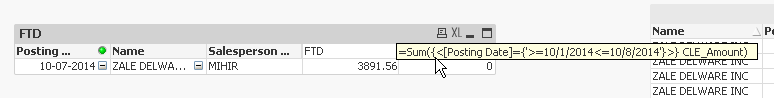# QlikView App Dev

Discussion Board for collaboration related to QlikView App Development.

Announcements
An issue has been identified on Qlik Cloud hub, please visit our Status Update Page for details: GET THE LATEST
cancel
Showing results for
Did you mean:Creator II

## need help??

Hi Community,

how to calculate MTD and YTD

MTD:

=Sum({<[Posting Date]={'>=\$(=MonthStart(Today()))<=\$(=Today())'}>} CLE_Amount)

i am using this expression.. but unable to get values..

6 RepliesPartner - Champion III

Sum({<[Posting Date]={'\$(>=MonthStart(Today())<=Today())'}>} CLE_Amount)

the \$(= ... must be applied to the whole sub expression

let me knowMaster III

Hi Paul,

format of Posting Date is DD-MM-YYYY, but in script your DateFormat is D/M/YYYY

You can try

....\$(=Date(MonthStart(Today()),'DD-MM-YYYY')))

also in \$(=Today())

Regards,
AntonioCreator II
Author

Hi ,

Still i am unable to get values...Master II

Hi Paul,

try like this:

SUM({<Date = , Month =, Quarter = , Date = {">=\$(=MonthStart(Today()))<=\$(=Today())"}>}Sales)

will give you Total Sales for Current Month to Date

SUM({<Date = , Month =, Quarter = , Date = {">=\$(=YearStart(Today()))<=\$(=Today())"}>}Sales)

will give you Total Sales For Current Year to Date

Regards

KC

Best Regards,
KCMaster III

See AttachmentMVP

it seems you have different format for the dates, in dimension and in set analysis expressiontry with

=Sum({<[Posting Date]={">=\$(=date(MonthStart(Today()),'MM-DD-YYYY'))<=\$(=date(Today(),'MM-DD-YYYY'))"}>} CLE_Amount)Community Browser